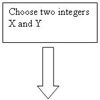#### You may also like### Route to Root

A sequence of numbers x1, x2, x3, ... starts with x1 = 2, and, if you know any term xn, you can find the next term xn+1 using the formula: xn+1 = (xn + 3/xn)/2 . Calculate the first six terms of this sequence. What do you notice? Calculate a few more terms and find the squares of the terms. Can you prove that the special property you notice about this sequence will apply to all the later terms of the sequence? Write down a formula to give an approximation to the cube root of a number and test it for the cube root of 3 and the cube root of 8. How many terms of the sequence do you have to take before you get the cube root of 8 correct to as many decimal places as your calculator will give? What happens when you try this method for fourth roots or fifth roots etc.?### Divided Differences

When in 1821 Charles Babbage invented the `Difference Engine' it was intended to take over the work of making mathematical tables by the techniques described in this article.### Procedure Solver

Can you think like a computer and work out what this flow diagram does?

# Ip?

##### Age 16 to 18Challenge Level

Have you managed to solve the entire Stage 5 Cipher Challenge? Solutions are now closed, but perhaps you want to take up the full challenge.

Patrick from Woodbridge School, England was the first to solve this cipher
Joseph from Hong Kong was the only other successful solver.

The solution is

Congratulations if you can read this, this encryption's getting quite complicated. The vigenere method of encryption commutes with a transposition if its row and column lengths are one more than a multiple of the keyword length, so in this case with a keyword of length two we need them to both be odd. I hope you've enjoyed decrypting these messages, why not try coming up with some ciphers yourself and testing your friends.

vigenere with keyword pi
transposition